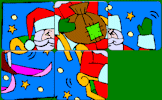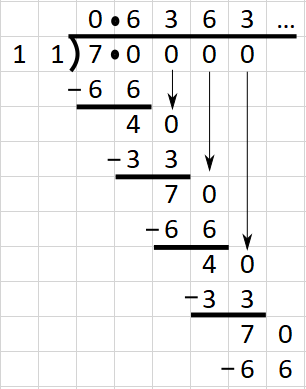# Recurring Decimals

## Change recurring decimals into their corresponding fractions and vica versa.

##### Level 1Level 2Exam-StyleHelpMore Decimals

Do not use a calculator. You can earn a trophy if you get at least 9 questions correct and you do this activity online.

1. Convert the recurring decimal $$0.\dot 6$$ to a fraction in its simplest form.

Working:2. Convert the recurring decimal $$0.\dot 4$$ to a fraction in its simplest form.

Working:3. Convert the recurring decimal $$0.\dot 5$$ to a fraction in its simplest form.

Working:4. The decimal $$0.\dot 7\dot 2$$ has a period of length two. Convert it to a fraction in its simplest form.

Working:5. The decimal $$0.\dot 6\dot 3$$ also has a period of length two. Convert it to a fraction in its simplest form.

Working:6. Convert the recurring decimal $$0.1\dot 6$$ to a fraction in its simplest form.

Working:7. Convert the recurring decimal $$0.91\dot 6$$ to a fraction in its simplest form.

Working:8. The decimal $$0.2\dot 2\dot 7$$ has a period of length two. Convert it to a fraction in its simplest form.

Working:9. Calculate $$2 \times$$ $$0.\dot 1\dot 8$$ giving your answer as a fraction in its lowest terms.

Working:10. Calculate $$1.5 \times$$ $$0.\dot 4\dot 5$$ giving your answer as a fraction in its lowest terms.

Working:11. Calculate ($$0.08\dot 3$$)2 giving your answer as a fraction in its lowest terms.

Working:12. Calculate $$\sqrt{0.\dot 4}$$ giving your answer as a fraction in its lowest terms.

Working:Check

This is Recurring Decimals level 2. You can also try:
Level 1

## Instructions

Try your best to answer the questions above. Type your answers into the boxes provided leaving no spaces. As you work through the exercise regularly click the "check" button. If you have any wrong answers, do your best to do corrections but if there is anything you don't understand, please ask your teacher for help.

When you have got all of the questions correct you may want to print out this page and paste it into your exercise book. If you keep your work in an ePortfolio you could take a screen shot of your answers and paste that into your Maths file.## More Activities:

Mathematicians are not the people who find Maths easy; they are the people who enjoy how mystifying, puzzling and hard it is. Are you a mathematician?

Comment recorded on the 24 May 'Starter of the Day' page by Ruth Seward, Hagley Park Sports College:

"Find the starters wonderful; students enjoy them and often want to use the idea generated by the starter in other parts of the lesson. Keep up the good work"

Comment recorded on the 1 February 'Starter of the Day' page by M Chant, Chase Lane School Harwich:

"My year five children look forward to their daily challenge and enjoy the problems as much as I do. A great resource - thanks a million."

Each month a newsletter is published containing details of the new additions to the Transum website and a new puzzle of the month.

The newsletter is then duplicated as a podcast which is available on the major delivery networks. You can listen to the podcast while you are commuting, exercising or relaxing.

Transum breaking news is available on Twitter @Transum and if that's not enough there is also a Transum Facebook page.

#### ChristMathsChristmas activities make those December Maths lessons interesting, exciting and relevant. If students have access to computers there are some online activities to keep them engaged such as Christmas Ornaments and Christmas Light Up.

There are answers to this exercise but they are available in this space to teachers, tutors and parents who have logged in to their Transum subscription on this computer.

A Transum subscription unlocks the answers to the online exercises, quizzes and puzzles. It also provides the teacher with access to quality external links on each of the Transum Topic pages and the facility to add to the collection themselves.

Subscribers can manage class lists, lesson plans and assessment data in the Class Admin application and have access to reports of the Transum Trophies earned by class members.

Subscribe

## Go Maths

Learning and understanding Mathematics, at every level, requires learner engagement. Mathematics is not a spectator sport. Sometimes traditional teaching fails to actively involve students. One way to address the problem is through the use of interactive activities and this web site provides many of those. The Go Maths page is an alphabetical list of free activities designed for students in Secondary/High school.

## Maths Map

Are you looking for something specific? An exercise to supplement the topic you are studying at school at the moment perhaps. Navigate using our Maths Map to find exercises, puzzles and Maths lesson starters grouped by topic.

## Teachers

If you found this activity useful don't forget to record it in your scheme of work or learning management system. The short URL, ready to be copied and pasted, is as follows:

Alternatively, if you use Google Classroom, all you have to do is click on the green icon below in order to add this activity to one of your classes.

It may be worth remembering that if Transum.org should go offline for whatever reason, there is a mirror site at Transum.info that contains most of the resources that are available here on Transum.org.

When planning to use technology in your lesson always have a plan B!

Transum,

Wednesday, August 30, 2017

What is the difference between $$0.\dot 9$$ and one?
"

Ann Roberts, London

Sunday, September 27, 2020

"Most fractions convert to recurring (repeating) decimals. Think of ⅓ converting to 0.333...

There are some fractions that convert to terminating decimals. Think of ½ converting to 0.5.

Which fractions convert to terminating decimals? I'll tell you. Write your fraction in its lowest terms n/d. Calculate the prime factorisation of the denominator d. Your fraction will only convert to a terminating decimal if: the prime factorisation of the denominator d contains ONLY 2s and/or 5s. In other words, the prime factorisation might include only 2s, or only 5s or a combinations of 2s and 5s. No other number is allowed.

Let me show you. 1/20 converts to a terminating decimal because the prime factorisation of 20 is 2x2x5 (so only 2s and 5s).

1/9 converts to a recurring decimal because the prime factorisation of 9 is 3x3. (3s are not allowed).

Use this rule to see if you can find some more fractions that convert to terminating decimals."

Do you have any comments? It is always useful to receive feedback and helps make this free resource even more useful for those learning Mathematics anywhere in the world. Click here to enter your comments.For All:

## Help

A decimal with a repeating digit (or set of digits) is called a recurring decimal.

For example $$0.77777777...$$ is a recurring decimal and is called "nought point seven recurring"

$$9.247347347...$$ is also a recurring decimal and is called "nine point two four seven recurring"

The period of a recurring decimal is the number of digits in the repeating section so for the second example above the period is three.

A more efficient way of writing out a recurring decimal is by only writing the repeating digit once but putting a dot over the first and last number in the repeating sequence. Another method is drawing a line over the repeating digit or digits.Here are some examples.

$$0.333333333... = 0.\dot 3 = 0.\overline 3$$

$$0.76531531531... = 0.76\dot 53\dot 1 = 0.76\overline{531}$$

A fraction can be converted to a decimal using long division; dividing the numerator by the denominator. If the decimal is recurring the repeating pattern of numbers will be spotted in the long division working. The following example shows the repeating patterns when converting $$\frac{7}{11}$$ to a decimal:There are two common methods for converting a recurring decimal to a fraction:

### Method 1

#### 1 repeating digit

Let the recurring decimal be represented by $$x$$

$$x = 0.8888888...$$

Multiply both sides by 10 (as there is one repeating digit)

$$10x = 8.8888888...$$

Subtract the first equation from the second

$$9x = 8$$ $$x = \frac{8}{9}$$

#### 2 repeating digits

Let the recurring decimal be represented by $$x$$

$$x = 1.36363636...$$

Multiply both sides by 100 (as there are two repeating digits)

$$100x = 136.36363636...$$

Subtract the first equation from the second

$$99x = 135$$ $$x = \frac{135}{99}$$ $$x = \frac{15}{11}$$

#### 3 repeating digits

The method is the same but multiply both sides by 1000.

### Method 2

#### 1 repeating digit

Example: convert $$0.8888888...$$ to a fraction.

This method requires you to know that $$\frac19 = 0.1111111...$$

$$0.8888888...$$ is exactly eight times $$0.1111111...$$

$$\therefore 0.8888888... = \frac{8}{9}$$

#### 2 repeating digits

Example: convert $$0.45454545$$ to a fraction in its lowest terms.

This method requires you to know that $$\frac{1}{99} = 0.01010101...$$

$$0.45454545...$$ is exactly forty five times $$0.01010101...$$

$$\therefore 0.45454545... = \frac{45}{99}$$ $$0.45454545... = \frac{5}{11}$$

#### 3 repeating digits

Example: convert $$0.\dot 61\dot 2$$ to a fraction in its lowest terms.

This method requires you to know that $$\frac{1}{999} = 0.\dot 00\dot 1$$

$$0.\dot 61\dot 2$$ is exactly six hundred and twelve times $$0.\dot 00\dot 1$$

$$\therefore 0.\dot 61\dot 2 = \frac{612}{999}$$ $$0.\dot 61\dot 2 = \frac{68}{111}$$

Don't wait until you have finished the exercise before you click on the 'Check' button. Click it often as you work through the questions to see if you are answering them correctly. You can double-click the 'Check' button to make it float at the bottom of your screen.

Answers to this exercise are available lower down this page when you are logged in to your Transum account. If you don’t yet have a Transum subscription one can be very quickly set up if you are a teacher, tutor or parent.

Close